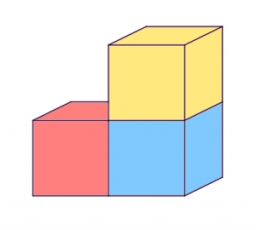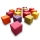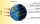# A plasticine

Jožko modeled from plasticine. He used 27g of plasticine to model a 3 cm long cube. How many grams of plasticine will it need to mold cubes with an edge of 6cm?

Result

m2 =  216 g

#### Solution:

$m_{1}=27 \ \text{g} \ \\ a=3 \ \text{cm} \ \\ V_{1}=a^3=3^3=27 \ \text{cm}^3 \ \\ \ \\ b=6 \ \text{cm} \ \\ V_{2}=b^3=6^3=216 \ \text{cm}^3 \ \\ \ \\ m_{2}=m_{1} \cdot \ \dfrac{ V_{2} }{ V_{1} }=27 \cdot \ \dfrac{ 216 }{ 27 }=216 \ \text{g}$Our examples were largely sent or created by pupils and students themselves. Therefore, we would be pleased if you could send us any errors you found, spelling mistakes, or rephasing the example. Thank you!

Leave us a comment of this math problem and its solution (i.e. if it is still somewhat unclear...):Be the first to comment!Tips to related online calculators
Tip: Our volume units converter will help you with the conversion of volume units.
Tip: Our Density units converter will help you with the conversion of density units.

## Next similar math problems:

1. An exampleAn example is playfully for grade 6 from Math and I don't know how to explain it to my daughter when I don't want to use the calculator to calculate the cube root. Thus: A cuboid was made from a block of 16x18x48 mm of modeline. What will be the edge of
2. Tetrahedral pyramid 8Let’s all side edges of the tetrahedral pyramid ABCDV be equally long and its base let’s be a rectangle. Determine its volume if you know the deviations A=40° B=70° of the planes of adjacent sidewalls and the plane of the base and the height h=16 of the p
3. Two rectangular boxesTwo rectangular boxes with dimensions of 5 cm, 8 cm, 10 cm, and 5 cm, 12 cm, 1 dm are to be replaced by a single cube box of the same cubic volume. Calculate its surface.
4. The edge of a cubeHow much does the edge of a cube of 54.9 cm3 measure?
5. Cuboid and ratioFind the dimensions of a cuboid having a volume of 810 cm3 if the lengths of its edges coming from the same vertex are in ratio 2: 3: 5
6. Wall thicknessThe hollow metal ball has an outside diameter of 40 cm. Determine the wall thickness if the weight is 25 kg and the metal density is 8.45 g/cm3.
7. Growth of woodThe annual growth of wood in the forest is estimated at 2%. In how many years will make the forest volume double?
8. Free space in the gardenThe grandfather's free space in the garden was in the shape of a rectangular triangle with 5 meters and 12 meters in length. He decided to divide it into two parts and the height of the hypotenuse. For the smaller part creates a rock garden, for the large
9. Sum of the seventeen numbersThe sum of the 17 different natural numbers is 154. Determine the sum of the two largest ones.
10. Triangular prismThe base of the perpendicular triangular prism is a rectangular triangle with a hypotenuse of 10 cm and one leg of 8 cm. The prism height is 75% of the perimeter of the base. Calculate the volume and surface of the prism.
11. Block or cuboidThe wall diagonals of the block have sizes of √29cm, √34cm, √13cm. Calculate the surface and volume of the block.
12. Squares ratioThe first square has a side length of a = 6 cm. The second square has a circumference of 6 dm. Calculate the proportions of the perimeters and the proportions of the contents of these squares? (Write the ratio in the basic form). (Perimeter = 4 * a, conte
13. Tropics and polar zonesWhat percentage of the Earth’s surface lies in the tropical, temperate and polar zone? Individual zones are bordered by tropics 23°27' and polar circles 66°33'
14. Volume of woodEvery year, at the same time, an increase in the volume of wood in the forest is measured. The increase is regularly p% compared to the previous year. If in 10 years the volume of wood has increased by 10%, what is the number p?
15. Power line poleFrom point A, the power line pole is seen at an angle of 18 degrees. From point B to which we get when going from point A 30m away from the column at an angle of 10 degrees. Find the height of the power pole.Calculate the volume (V) and the surface (S) of a regular quadrilateral prism whose height is 28.6 cm and the deviation of the body diagonal from the base plane is 50°.The regular triangular prism, whose edges are identical, has a surface of 2514 cm ^ 2 (square). Find the volume of this body in cm3 (l).